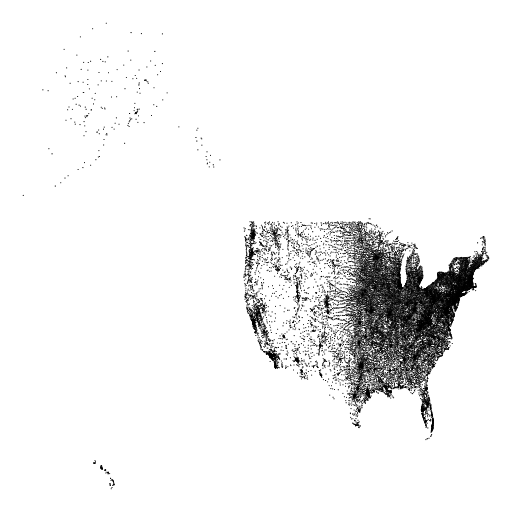HW1 : Plot Zip CodesAssignment: Read from the data file provided below. Take the Latitudes and Longitudes of each zip code and plot a point using the StdDraw package from Sedgewick plot all the Latitudes and Longitudes as points. StdDraw is contained in this jar file: stdlib.jar.

In order to read a file line by line you can do the following:

BufferedReader input = new BufferedReader(new FileReader(new File("zips.csv")));
String line;
line = input.readLine(); // this will be null when we reached the end of file


In order to parse a string you can do the following:

Scanner scan = new Scanner(line);
scan.useDelimiter(", *"); //tell the Scanner to split on commas
String first = scan.next();
String second = scan.next(); // this is the string following the first comma
double third = scan.nextDouble(); //find the next token that is a double
...


You can use StdDraw as follows. You will have to find the left, right, top, and bottom of where the points will be plotted. This is required in order to set the StdDraw bounds so the points will be visible.

// set bounds. These must be set before you plot any points
StdDraw.setXscale(leftbound,rightbound);
StdDraw.setYscale(bottombound,topbound);
// draw some points
StdDraw.point(1, 5);
StdDraw.point(5.12, 99);
// show the plot
StdDraw.show();


The data file: zips.csv is formated as follows:

IGNORE,ZIPCODE,STATE ,CITY    ,LAT      ,LNG      ,IGNORE,IGNORE
"25"  ,"02108","MA"  ,"BOSTON",71.068432,42.357603,3697  ,0.000614 

In this file the Latitude should be negative. In order to plot the map correctly you must make this value negative or you will see a mirror image.

Hints:

• Use System.out.println() to figure out what you are reading in
• Change the data so you are only plotting one state to make development easier
• Use Google Maps to figure out if your coordinates are correct
• Run one loop to find the boundaries of the map and another loop to plot them
• Read the file into GPSLocation objects and store them in an ArrayList

Deliverables: Your project should be turned in on the UNIX system inside your cs210 folder in a folder called hw1. Your program should do something like this: http://youtu.be/d7iAAS2QrH4. Your program should run with the following command:

//compile
javac -cp stdlib.jar PlotZipCodes.java
//run
java -cp .:stdlib.jar PlotZipCodes